×

Search anything:

# Scaled Dot-Product Attention

#### Deep Learning Machine Learning (ML)Get this book -> Problems on Array: For Interviews and Competitive Programming

Attention Mechanism have revolutionized the way NLP & Deep Learning models function by introducing the ability to mimic cognitive attention the way humans do in order to make predictions. There are several approaches to achieve this and this article at OpenGenus aims to walk you through the core operations of one of the most efficient approaches: The Scaled Dot-Product Attention technique.

1. What is Attention Mechanism?
2. First Matrix Multiplication.
3. Scaling of Matrices.
4. SoftMax Function.
5. Second Matrix Multiplication.

## What is Attention Mechanism?

An Attention Model is a technique used in Deep Learning applications that processes an input data and focuses on the more relevant input features based on the context by calculating the attention weights for each input feature based on its relevance to its current task. It was first used in NLP to improve the performance of Machine Translation tasks. The idea behind Attention Mechanism is inspired by human cognitive attention where just as we focus on certain parts of an image or text to process information, the model focuses on certain parts of the input data to make predictions.

Consider a sentence 'Bill is going to the theatre to watch a new movie'. The model processes this sentence and pairs each word with another word to find the context similarity between the words. The context similarity can be found out by generating a similarity matrix. The pairs 'watch' & 'movie', 'theatre' & 'watch' have relatively higher similarity than other pairs as it provides majority of the context.

Scaled Dot-Product Attention is one of the methods of Attention Mechanism. It was introduced in the paper 'Attention Is All You Need' which used Attention Mechanism in a feedforward network. Scaled dot product attention is a type of attention mechanism used in deep learning models, particularly in natural language processing (NLP) and computer vision. It is an efficient way to calculate the relevance between a query and a set of key-value pairs.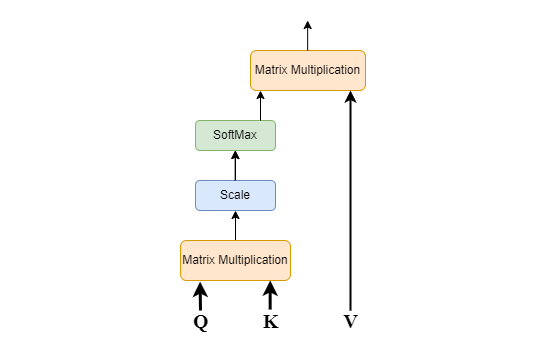Q-Query Vector of size d.
K-Key Vector of size d.
V-Value Vector of size d.

The size of both the Query and the Key vector should always be the same. However, the size of the Value Vector can be the same or different. In this case, the size of all the vectors is the same for simplicity.

Consider the previously mentioned input sequence which has 11 input tokens. Since there are 11 tokens in the sequence, the sequence length is 11. The feature vectors Xi to x11 are obtained by inputting the sequence in an Embedding Layer prior to inputting them in an Attention Layer.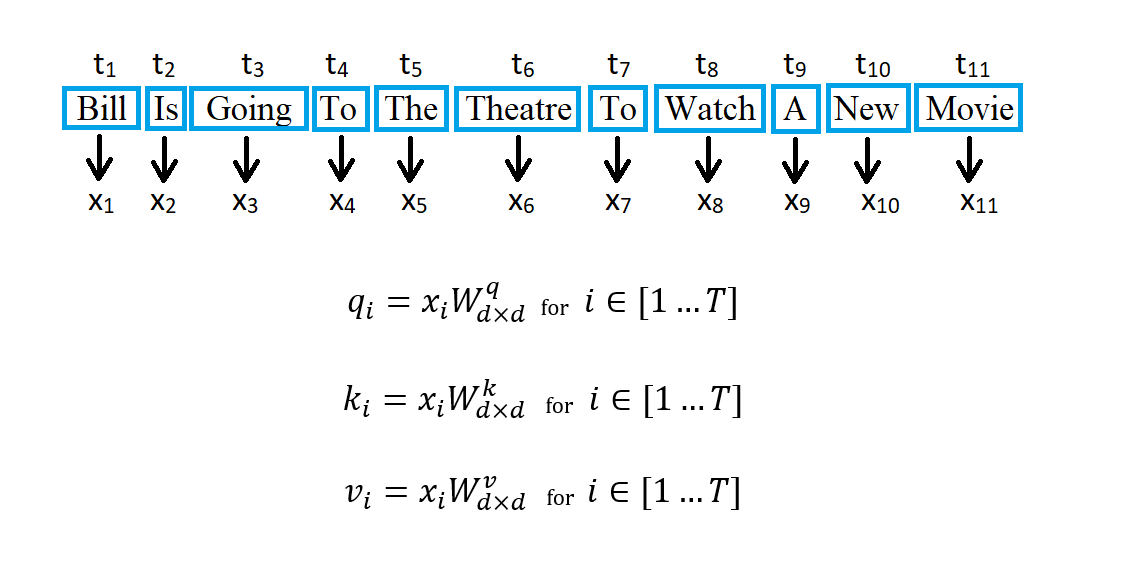Concatenating these feature vectors gives a matrix of size Txd where T is the sequence length. Then we perform matrix multiplication with learnable weights Wq, Wk and Wv.

## First Matrix Multiplication

The First Matrix Multiplication computes the similarity between the Query Matrix and the Key Matrix by performing a dot product operation. For performing a dot product, the column value of the first vector (Query Vector in this case) should be equal to the row value of the second vector (Key Vector). Hence, the key vector is transposed. After the multiplication, the compatibility matrix is obtained.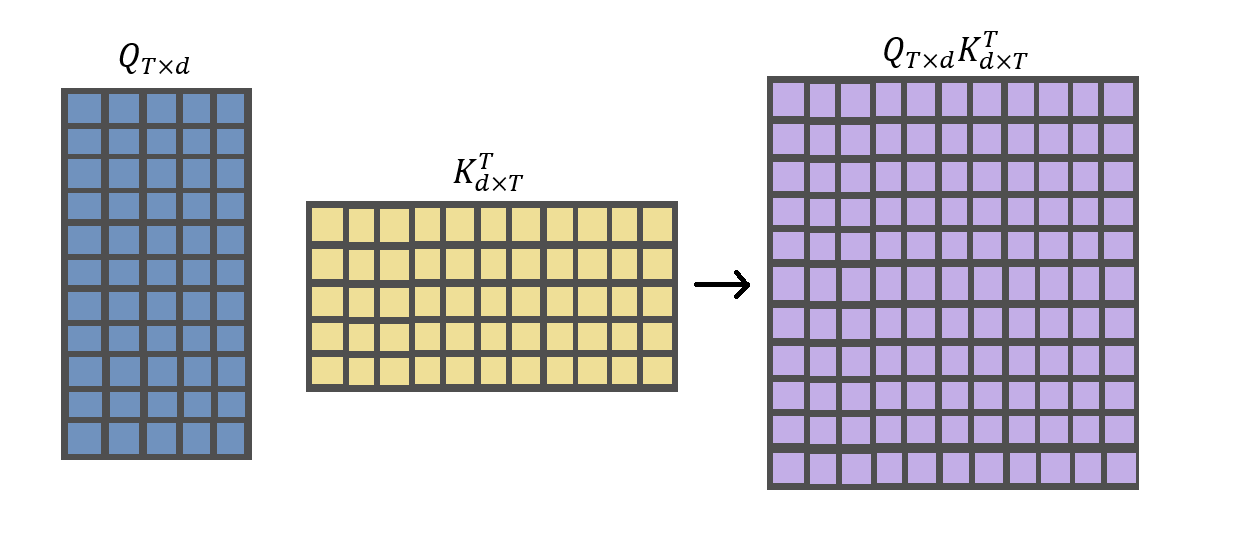## Scaling of Matrices

There are mainly two types of calculating Attention, i.e. Additive Attention and Multiplicative Attention. Multiplicative Attention is comparatively more efficient than Additive and both of them are fairly similar when 'd' is small. However, when the dimension d is large, Dot-Product attention performs poorly when compared to Additive Attention. This is due to large variance and leads to the Vanishing Gradients Problem.

The vanishing gradients problem is a phenomenon that can occur during the training of deep neural networks. It happens when the gradients of the loss function with respect to the weights of the network become very small as they are propagated backward through the layers of the network during the backpropagation algorithm. The is because the gradients are multiplied by the weights of each layer as they propagate backward, and small gradients can quickly become even smaller when multiplied by small weights.

Scaling of the matrix helps to stabilize the gradients during training. We have to scale the dot product of Q and K by 1/(square root of d) as shown below.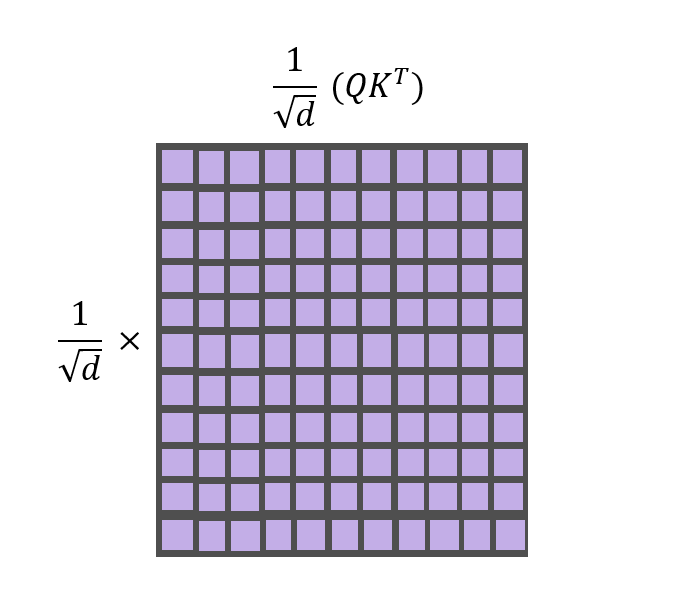## SoftMax Function

The SoftMax function normalizes the scores across all keys for a given query, ensuring that the attention weights sum up to 1. It is a mathematical function that is often used in machine learning to convert a set of numbers into a probability distribution. The SoftMax function is a critical component of the scaled dot product attention mechanism, as it allows the model to learn which parts of the input are most relevant to the current task. The function can be defined as: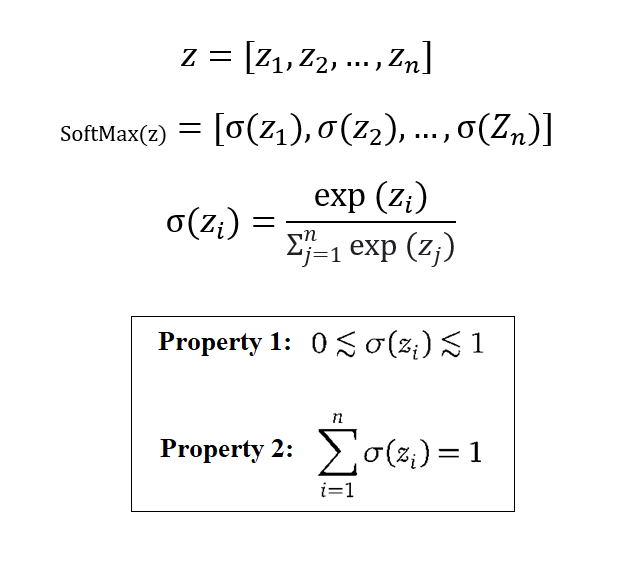Now we apply SoftMax to each row of the scaled compatibility matrix which means that in the output, the sum of each row will be 1. The obtained matrix is the Attention Matrix.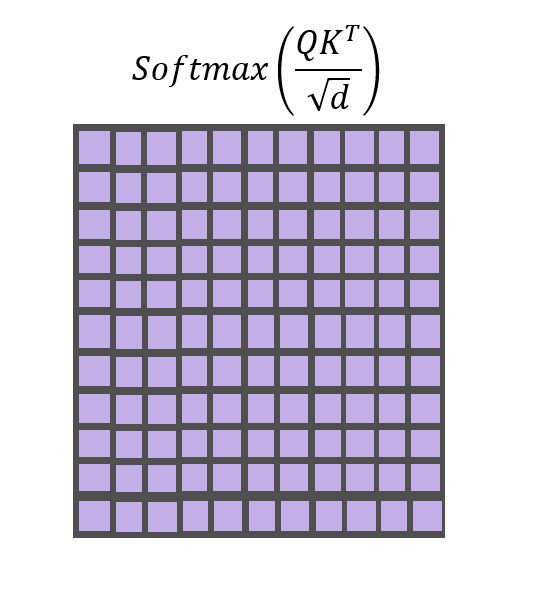## Second Matrix Multiplication

In the last step, we take the Attention Matrix A of dimensionality TxT that we obtained in the last step and perform Matrix Multiplication with Value Matrix of dimensionality Txd. After the matrix multiplication, we obtain the final matrix of dimensionality Txd which is the final output of the Attention Layer.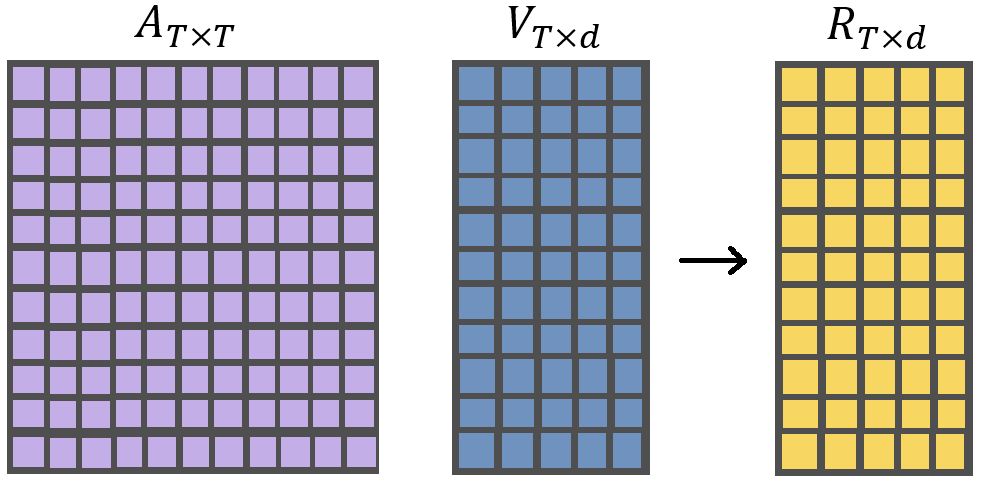The output of scaled dot product attention is a weighted sum of the values, where the weights are determined by the similarity between the query and each key. The output of scaled dot product attention is then typically fed into another layer of the neural network as part of the overall computation.

With this article at OpenGenus, you must have a comprehensive idea of the core operations involved in Scaled Dot-Product Attention.#### Ielin Daisy

Hi, I'm Ielin! I am a Computer Science undergraduate and an aspiring Game Developer. Currently developing my skills in Mobile Development. Love gaming, reading and making new connections.